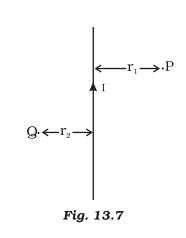# AB is a current-carrying conductor in the plane of the paper as shown in the figure. What are the directions of magnetic fields produced by it at points P and Q? Given r1 > r2, where will the strength of the magnetic field be larger?By applying the right-hand thumb rule, the direction of magnetic field would be anti-clockwise as shown in diagram around the direction of current. So, the magnetic field at point P would be into the plane of paper. At point Q, the direction of magnetic current would be out from the plane of paper.
The strength of the magnetic field is stronger near the conductor and weaker as we move away from the conductor as the strength of the conductor is inversely proportional to the distance from the conductor. Given that the distance of point P from the conductor is greater than the distance of point Q to the conductor, the magnetic field would be stronger near point Q than near point P.(57)(18)